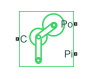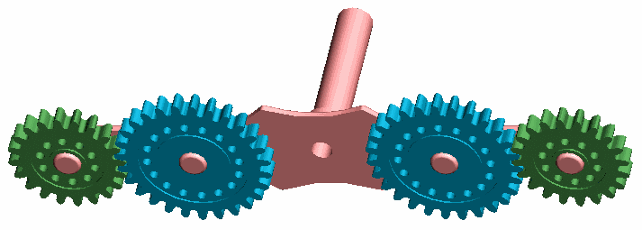# Planet-Planet

Planetary gear set of carrier, inner planet, and outer planet wheels with adjustable gear ratio and friction losses

• Library:
• Simscape / Driveline / Gears / Planetary Subcomponents

•## Description

The Planet-Planet gear block represents a carrier and two inner-outer planet gear couples. Both planet gears are connected to and rotate with respect to the carrier. The planet gears corotate with a fixed gear ratio that you specify. For model details, see Equations.### Thermal Model

You can model the effects of heat flow and temperature change by enabling the optional thermal port. To enable the port, set Friction model to ```Temperature-dependent efficiency```.

### Equations

Ideal Gear Constraints and Gear Ratios

The Planet-Planet block imposes one kinematic and one geometric constraint on the three connected axes:

`${r}_{\text{C}}{\omega }_{\text{C}}={r}_{\text{Po}}{\omega }_{\text{Po}}+{r}_{\text{Pi}}{\omega }_{\text{Pi}}$`
`${r}_{\text{C}}={r}_{\text{Po}}+{r}_{\text{Pi}}$`

The outer planet-to-inner planet gear ratio is

`${g}_{\text{oi}}={r}_{\text{Po}}/{r}_{\text{Pi}}={N}_{\text{Po}}/{N}_{\text{Pi}},$`

where N is the number of teeth on each gear. In terms of this ratio, the key kinematic constraint is

The three degrees of freedom reduce to two independent degrees of freedom. The gear pair is (1, 2) = (Pi,Po).

The torque transfer is

In the ideal case where there is no torque loss, τloss = 0.

Nonideal Gear Constraints and Losses

In the nonideal case, τloss ≠ 0. For more information, see Model Gears with Losses.

### Variables

Use the Variables settings to set the priority and initial target values for the block variables before simulating. For more information, see Set Priority and Initial Target for Block Variables.

### Assumptions and Limitations

• Gear inertia is assumed to be negligible.

• Gears are treated as rigid components.

• Coulomb friction slows down simulation. For more information, see Adjust Model Fidelity.

## Ports

### Conserving

expand all

Rotational mechanical conserving port associated with the planet gear carrier.

Rotational mechanical conserving port associated with the outer planet gear.

Rotational mechanical conserving port associated with the inner planet gear.

Thermal conserving port associated with heat flow. Heat flow affects the power transmission efficiency by altering the gear temperatures.

#### Dependencies

To enable this port, set Friction model to `Temperature-dependent efficiency`.

## Parameters

expand all

### Main

Ratio, goi, of the outer planet gear to inner planet gear rotations as defined by the number of outer planet gear teeth divided by the number of inner planet gear teeth. This gear ratio must be strictly positive.

### Meshing Losses

Friction model for the block:

• `No meshing losses - Suitable for HIL simulation` — Gear meshing is ideal.

• `Constant efficiency` — Transfer of torque between the gear wheel pairs is reduced by a constant efficiency, η, such that 0 < η ≤ 1.

• `Temperature-dependent efficiency` — Transfer of torque between the gear wheel pairs is defined by the table lookup based on the temperature.

Torque transfer efficiency, ηPP, for the outer and inner planet gear wheel pair meshing. This value must be in the range (0,1].

#### Dependencies

To enable this parameter, set Friction model to `Constant efficiency`.

Vector of temperatures used to construct a 1-D temperature-efficiency lookup table. The vector elements must increase from left to right.

#### Dependencies

To enable this parameter, set Friction model to `Temperature-dependent efficiency`.

Vector of output-to-input power ratios that describe the power flow from the outer planet gear to the inner planet gear, ηPP. The block uses the values to construct a 1-D temperature-efficiency lookup table.

Each element is an efficiency that relates to a temperature in the Temperature vector. The length of the vector must be equal to the length of the Temperature vector. Each element in the vector must be in the range (0,1].

#### Dependencies

To enable this parameter, set Friction model to ```Temperature-dependent efficiency```.

Power threshold, pth, above which full efficiency is in effect. Below this value, a hyperbolic tangent function smooths the efficiency factor.

When you set Friction model to `Constant efficiency`, the block lowers the efficiency losses to zero when no power is transmitted. When you set Friction model to `Temperature-dependent efficiency`, the block smooths the efficiency factors between zero when at rest and the values provided by the temperature-efficiency lookup tables at the power thresholds.

#### Dependencies

To enable this parameter, set Friction model to `Constant efficiency` or ```Temperature-dependent efficiency```.

### Viscous Losses

Viscous friction coefficient μPi for the inner planet-carrier gear motion.

### Thermal Port

To enable this parameter, set Friction model to `Temperature-dependent efficiency`.

Thermal energy required to change the component temperature by a single temperature unit. The greater the thermal mass, the more resistant the component is to temperature change.

#### Dependencies

To enable this parameter, set Friction model to ```Temperature-dependent efficiency```.

expand all

## Version History

Introduced in R2011a